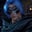Related Tags

typescript
javascript
communitycreator

# What are variables in TypeScript?Gutha Vamsi Krishna

A variable is a named location in memory to store data. In TypeScript, an extension of Javascript, there are three different keywords to define variables: var, let, and const.

Visual representation of variable in code

### var

var a = 11;

• The var keyword is used to define a variable and has scope outside the block in which it is used. This is known as var scoping or function scoping.
• Once we define var, we can access it anywhere in the program.
• var can be redeclared many times, but this may lead to bugs.
//var redeclaration example

function f(x:any) {

//declaring first time
var x:any = 1;
console.log(x);

//redeclaring second time
var x:any = 2;
console.log(x)
if (true) {

//redeclaring third time
var x:any = 3;
console.log(x)

}
}

//calling the function
f(0)

### let

let a = 11;

• The let keyword is used to define a variable whose scope is limited to the block it is defined in.
• If you run the code snippet below, you will get the error Cannot find name x because we define and declare x in the if block with the let keyword, so we cannot access it from outside the if block.
function f(isLoggedIn: boolean) {
//defining variable x with let keyword in if block
if (isLoggedIn) {
let x = 10;
}
return x;
}

//calling the function
f(true)
• To resolve the error in the code above, we need to define the variable x outside of the if block and inside the function with the let keyword so it has function scope.
• Check the code snippet below for the modified code.
function f(isLoggedIn: boolean) {
//defining variable x with let keyword in function block
let x;
if (isLoggedIn) {
x = 10;
}
return x;
}

//calling the function
console.log(f(true))

Unlike var, variables that are declared with let cannot be redeclared, as shown in the code below.

function f(){
let x = 5;

//redeclaring x
let x = 10;
return x;
}

f()

### const

const pi = 3.14

• const is a keyword that is used to declare variables where the value of the variable is restricted to change.
• const is useful when you want to define some constant values and you don’t want other programmers to change that value in the rest of the program.
const  pi = 3.14;

// changing const value throws error
pi = 1;

### Rules

• Variable names can contain alphabets and digits.
• Allowed special characters are _ and ＄.

Below are the example code snippets for variable rules.

//variable can contain alphabets and digits
var abc123 = "Example"
console.log(abc123)
//variable names cannot start with a digit
//It will throw error
var 4abc = "Example";
console.log(4abc);
//Allowed special character _ and \$.

var a_b = "Example";
console.log(a_b)

RELATED TAGS

typescript
javascript
communitycreator

CONTRIBUTORGutha Vamsi Krishna
RELATED COURSES

View all Courses

Keep Exploring

Learn in-demand tech skills in half the time